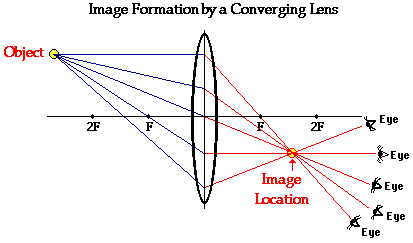Categories

# Applying the Three Rules of Refraction

we will investigate the method for drawing ray diagrams for objects placed at various locations in front of a double convex lens. To draw these ray diagrams, we will have to recall the three rules of refraction for a double convex lens:

• Any incident ray traveling parallel to the principal axis of a converging lens will refract through the lens and travel through the focal point on the opposite side of the lens.
• Any incident ray traveling through the focal point on the way to the lens will refract through the lens and travel parallel to the principal axis.
• An incident ray that passes through the center of the lens will in effect continue in the same direction that it had when it entered the lens.

Earlier in this lesson, the following diagram illustrating the path of light from an object through a lens to an eye placed at various locations was shown.In this diagram, five incident rays are drawn along with their corresponding refracted rays. Each ray intersects at the image location and then travels to the eye of an observer. Every observer would observe the same image location and every light ray would follow the Snell’s Law of refraction. Yet only two of these rays would be needed to determine the image location since it only requires two rays to find the intersection point. Of the five incident rays drawn, three of them correspond to the incident rays described by our three rules of refraction for converging lenses. We will use these three rays through the remainder of this lesson, merely because they are the easiest rays to draw. Certainly two rays would be all that is necessary; yet the third ray will provide a check of the accuracy of our process.